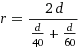## Example Questions

### Example Question #31 : Proportion / Ratio / Rate

Alex runs around his school race track one time in 15 minutes and takes another 25 minutes to run around a second time. If the course is 4 miles long, what is his approximate average speed in miles per hour for the entire run?

6

10

4

8

6

Explanation:

15 + 25 = 40 minutes. 40 minutes is 2/3 of an hour. Distance = rate x time. Using this formula, we have 4 = (2/3) r. To solve for r we multiply both sides by (2/3). r = 6

### Example Question #31 : Proportion / Ratio / Rate

If a car travels 60 mph for 2 hours, 55 mph for 1.5 hours and 30 mph for 45 minutes, how far has the car traveled?

202.5 miles

1552.5 miles

225 miles

120 miles

145 miles

225 miles

Explanation:

Distance traveled = mph x hour

60mph x 2hours + 55mph x 1.5 hours + 30 mph x 45 minutes (or .75 hours) =

120 miles + 82.5 miles + 22.5 miles = 225 miles

### Example Question #33 : Proportion / Ratio / Rate

If an object travels at 1200 ft per hour, how many minutes does it take to travel 180 ft?

10 minutes

9 minutes

8 minutes

7 minutes

11 minutes

9 minutes

Explanation:

1200 ft per hour becomes 20 ft per second (divide 1200 by 60 because there are 60 minutes in an hour). 180/20 is 9, giving 9 minutes to travel 180 ft.

### Example Question #11 : How To Find Rate

If you live 3 miles from your school. What average speed do you have to ride your bike get to your school from your house in 15 minutes?

12 miles/hour

10 miles/ hour

5 miles/hour

15 miles/hour

3 miles/hour

12 miles/hour

Explanation:

The best way to find speed is to divide the distance by time. Since time is given in minutes we must convert minutes to hours so that our units match those in the answer choices. (3miles/15min)(60min/1hr)=12miles/hr;  Remember when multipliying fractions to multiply straight across the top and bottom.

### Example Question #35 : Proportion / Ratio / Rate

If an airplane is flying 225mph about how long will it take the plane to go 600 miles?

2.7 hours

2.5 hours

3.5 hours

3.2 hours

2.4 hours

2.7 hours

Explanation:

Speed = distance /time; So by solving for time we get time = distance /speed. So the equation for the answer is (600 miles)/ (225 miles/hr)= 2.67 hours; Remember to round up when the last digit of concern is 5 or more.

### Example Question #36 : Proportion / Ratio / Rate

Vikki is able to complete 4 SAT reading questions in 6 minutes. At this rate, how many questions can she answer in 3 1/2 hours?

210

35

150

170

140

140

Explanation:

First, find how many minutes are in 3 1/2 hours: 3 * 60 + 30 = 210 minutes. Then divide 210 by 6 to find how many six-minute intervals are in 210 minutes: 210/6 = 35. Since Vikki can complete 4 questions every 6 minutes, and there are 35 six-minute intervals we can multiply 4 by 35 to determine the total number of questions that she can complete.

4 * 35 = 140 problems.

### Example Question #37 : Proportion / Ratio / Rate

The price of k kilograms of quartz is 50 dollars, and each kilogram makes s clocks. In terms of s and k, what is the price, in dollars, of the quartz required to make 1 clock?

(50k ) / s
(50s ) / k
(ks ) / 50
s / (50k )
50 / (ks )
Correct answer: 50 / (ks )
Explanation:

We want our result to have units of "dollars" in the numerator and units of "clocks" in the denominator. To do so, put the given information into conversion ratios that cause the units of "kilogram" to cancel out, as follows: (50 dollar/k kilogram)* (1 kilogram / s clock) = 50/(ks) dollar/clock.

Since the ratio has dollars in the numerator and clocks in the denominator, it represents the dollar price per clock.

### Example Question #38 : Proportion / Ratio / Rate

Minnie can run 5000 feet in 15 minutes.  At this rate of speed, how long will it take her to fun 8500 feet?

18.5 min

25.5 min

20 min

75.5 min

36.5 min

25.5 min

Explanation:

Find the rate of speed.  5000ft/15 min = 333.33 ft per min

Divide distance by speed to find the time needed

8500ft/333.33ft per min = 25.5

### Example Question #40 : Proportion / Ratio / Rate

Ruby drives to her grandmother's house and back. On the way there, she travels at an average speed of 40 miles per hour. On the way back, she travels at an average speed of 60 miles per hour. What is Ruby's average speed for the entire round trip?

40

48

60

50

52

48

Explanation:

Remember d=rt where d = distance, r = rate, and t = time.  In this case, the distance to and the distance from are the same, so we know that

d = rt1 and d = r2 t2

Since r1 = 40 and r2 = 60, we can solve for the times and get

t1 = d/40 and t2 = d/60

Average speed is found by total distance over total time, so we getMultiplying the numerator and denominator by 120/d we get 240/(3 + 2) = 48.

### Example Question #41 : Proportion / Ratio / Rate

If an object takes 10 minutes to go 3 miles, how fast is the object going?

3/10 mph

36 mph

1/2 mph

18 mph

10/3 mph